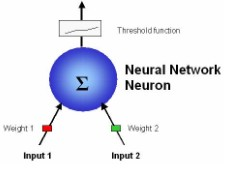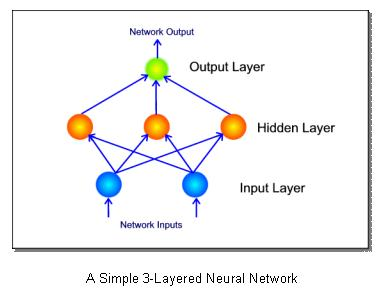index/CrySis/Research/HistoryAs their name implies, neural networks take a cue from the human brain by emulating its structure. Work on neural networks began in the 1940s by McCulloch and Pitts and was followed by the advent of Frank Rosenblatt’s Perceptron. The neuron is the basic structural unit of a neural network. In the brain, a neuron receives electrical impulses from numerous sources. If there are enough agonist signals, the neuron fires and triggers all of its outputs. A neural network neuron functions similarly. A neuron receives any number of inputs that possess weights based on their importance. Just as in a real neuron, the weighted inputs are summed and output based on a threshold function sent to every neuron downstream. A barrage of positive inputs will provide a positive output and visa-versa. The original Perceptron received two inputs, and gave a single output. Although this system worked well for simple problems, Minsky demonstrated in 1969 that non-linear classifications, such as exclusive-or (XOR) logic, were impossible.It wasn’t until the 1980’s that training algorithms for multi-layered networks were introduced to solve this problem, restoring faith in neural networks. A multi-layered network consists of numerous neurons, which are arranged into levels. Each level is interconnected with the one above and below it. The first layer receives external inputs and is aptly named the input layer. The top layer provides the classification solution, and is called the output layer. Sandwiched between the input and output layers are any number of hidden layers. It is believed that a three-layered network can accurately classify any non-linear function. Multi-layered networks commonly use more sophisticated threshold functions such as the sigmoid function. This is advantageous because the sigmoid function’s range is [-0.5, 0.5] and therefore prevents any individual output from becoming too large and “overpowering” the network